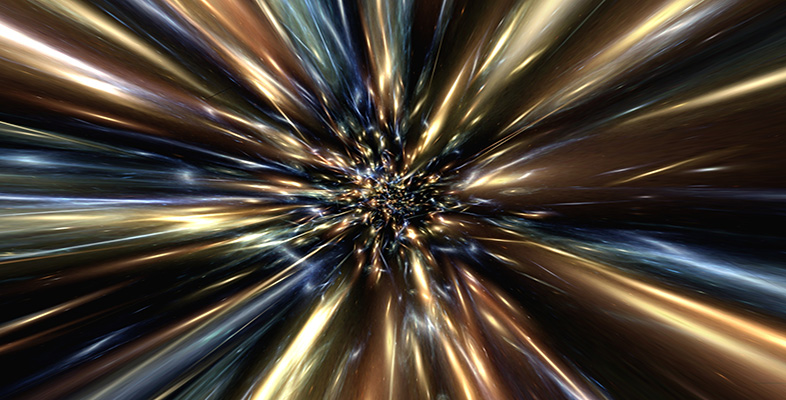The Big Bang

Start this free course now. Just create an account and sign in. Enrol and complete the course for a free statement of participation or digital badge if available.

Free course

# 7.3 The formation of light nuclei

It is the very high temperatures that make the early stages of the big bang relatively simple to calculate. When it comes to the formation of the first nuclei, we are looking at a temperature that has dropped to about 109 K, this being achieved approximately 3 minutes after the instant of the big bang. For nuclei to form, the temperature must still be high enough that charged nuclei can approach each other closely – despite the electrostatic repulsion between their positive charges – thus allowing further fusion to take place. On the other hand, if the temperature is too high, any nuclei that are formed will immediately be disrupted again by a subsequent violent collision with another particle.

The only particles taking part (to any significant extent) in the reactions at this time were neutrons, protons, electrons, and photons. In addition there were neutrinos – a very weakly interacting particle. The simplest reaction, and perhaps the most important as far as cosmology is concerned, is the fusion of a neutron (n) and a proton (p) to give a deuteron, d (i.e. a deuterium nucleus) plus a photon of sufficiently high energy to be called a gamma ray ():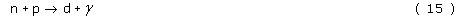Note that the energy is mostly carried away by the gamma ray. This is essential if the neutron and proton are to bind, for otherwise the neutron and proton would simply bounce apart again. The probability of this fusion reaction occurring is high, as is that of the reverse reaction, in which a gamma ray destroys a deuteron.

As we have noted, significant numbers of deuterons could form and remain undisturbed only after the temperature had dropped to about 109 K. On the other hand, by the time the temperature had dropped to about 5 × 108 K, at 20 minutes after the big bang, the density had become so reduced that there were now no longer sufficient collisions taking place to produce many deuterons. Deuteron formation was therefore confined to the period during which the temperature fell between these two values.

More complicated nuclei can be produced by similar reactions. For example, helium (4He) can be formed through the fusion of two deuterons: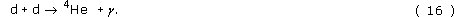But there are also several other ways in which it can be formed, each involving two steps. For example: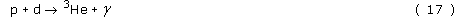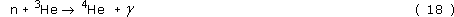and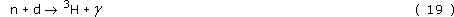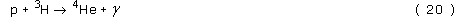where 3H is a tritium nucleus (a triton), i.e. the nuclear isotope of hydrogen consisting of a proton and two neutrons.

Each of the above reactions can occur in either direction. Thus, for example, corresponding to Reaction 20 there will be its reverse: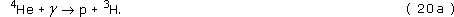Given sufficient time, an equilibrium state would arise whereby the particles present will yield the same rate for Reaction 20a as for Reaction 20. And the same will apply for each of the other reactions. Thus, corresponding to any particular temperature (the temperature governing the reaction rates) it is possible to estimate the proportions of the various particles that would be present once equilibrium had been established. This estimate is independent of whether equilibrium was reached quickly (under conditions of high density where the interactions happen frequently) or slowly (under low-density conditions).

So much for the density-independent equilibrium state achieved over a lengthy period of time. But the case of the big bang is different. The matter was rapidly dispersed only a short time after the mixture of neutrons and protons had started the chain of fusion processes. Under these circumstances, the final abundances of nuclides depended on how many collisions were able to take place before the dispersion effectively brought the processes to a halt. This in turn means that the final mix of particles is expected to depend on the density. More specifically, it is encounters with protons and neutrons that are significant, so it is the cosmic density of protons, neutrons and matter based on protons and neutrons that is important. This particular contribution to the overall density of the Universe is known as the baryonic density, since the proton and neutron are the lightest members of a family of particles known as baryons. We shall represent the average mass density of baryonic matter in the Universe as ρb/c2, preserving the symbol ρb for the associated energy density of baryonic matter. Thus, the baryonic density prevailing at the time of nuclear synthesis had an important part to play in governing the relative abundances of the light elements formed.

Roughly speaking, you can think of the baryonic density as the density of ‘ordinary’ matter. You are essentially made of baryonic matter as are the Earth, the Sun and all familiar objects.

There is a second reason why the baryonic density was important. It arises from the fact that while these fusion reactions were taking place, free neutrons (those that had not yet been incorporated into nuclei) were decaying: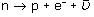where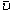is an antineutrino.

Unlike the fusion reactions, the rate for this decay is independent of baryonic density. Thus when the baryonic density changes, the balance between the two types of reaction changes. At low densities, a neutron travels further before colliding with another baryon, so it has a greater chance of decaying before being captured into a nucleus. Contrast this with collision reactions which have less probability of occurring at low densities. It is these different dependences on density that provide the second reason why the final mix of nuclei will depend on the baryonic density during the period of nuclear synthesis.

Now let us take a look at the result of detailed calculations. The rate of each reaction depends on the concentrations of the parent nuclei, on experimentally determined relationships between the reaction probability and the energies of the particles, and on the relationship between the equilibrium distribution of energies and the temperature. To find out the net effect of all the reactions is, mathematically, simply a matter of solving simultaneous differential equations; but they must be solved numerically, and judgement must be exercised in interpolating the experimental data. It is a lengthy computer calculation, even though it is basically straightforward. Several groups have made these calculations. We show the results of one particular investigation in Figure 31.

Figure 31 is based on a model with an assumed value of the baryonic density as it is today, ρb,now, from which one can extrapolate back in time to what it would have been at the time of the primordial nuclear synthesis (making due allowance for the expansion of the Universe between those two times). The mass fractions of the various nuclei were calculated as a function of time. Since the temperature at a given time can be calculated, the horizontal scales are marked with temperature (at the top) as well as time elapsed since the big bang (at the bottom).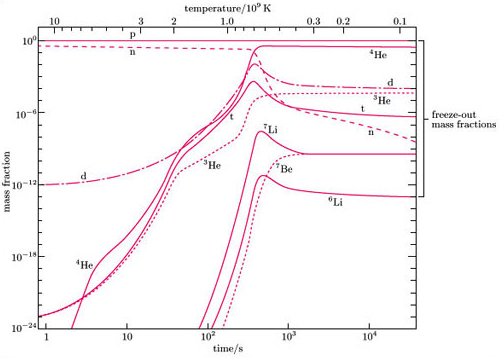from M.S. Smith, L.H. Kawano and R.A. Malaney (1993) The Astrophys. J. Supp., 85, 219–47 ©
from M.S. Smith, L.H. Kawano and R.A. Malaney (1993) The Astrophys. J. Supp., 85, 219–47
Figure 31 Evolution of nuclear abundances during the expansion of a typical model of the big bang. The vertical scale shows what fraction of the total mass the various types of nucleus form at different times. Note: H, He, Li and Be stand for the nuclei of hydrogen, helium, lithium and beryllium respectively, and the superscript indicates the mass of the isotope (e.g. 3He is the isotope of helium containing two protons and one neutron). The deuteron is signified by d, and the nucleus of tritium (two neutrons + one proton) by t.

Look first at the basic particles: neutrons and protons. Initially, at time 1 second, we have essentially 13% neutrons and 87% protons. This ratio can be calculated with confidence as it depends only on the mass difference between neutrons and protons.

As far as we are concerned, the interesting action starts just above 109 K, the temperature at which the proton and neutron numbers begin to drop because they are being used up in thermonuclear reactions (similar numbers of neutrons and protons are used up – it is only the logarithmic scale that makes the drop in the proton curve almost invisible). Some elements, such as helium (both the normal 4He and its isotope 3He), show no decrease, with the main production close to 109 K. The deuterium concentration, on the other hand, increases rapidly at temperatures around 109 K, but later (below 6 × 108 K) the concentration falls a little, because more deuterium is being used in making helium than is being synthesised from raw neutrons and protons. The point is that at this stage we are dealing with periods of time comparable to the mean lifetime of the neutron (930 s, i.e. about 15 minutes). Neutrons are being removed by decay – note the steady decline in its curve – and are thus no longer available for synthesising deuterons. However, there are still collisions going on that are destroying the deuterons. From a cosmologist's point of view, this is the crucial stage. The greater the baryonic density of the Universe at that time, the longer the process of deuteron destruction can continue after the synthesis of deuterons has effectively ceased, and therefore, the lower the final concentration of deuterium. It is true that this effect is partly offset by the fact that in a denser Universe, more deuterium would have been formed in the first place. But the destruction of deuterium is more sensitive to density than is its initial formation.

Below about 4 × 108 K, all the nuclide mass fractions – apart from the decaying neutrons and tritons, the latter decaying to 3He – are more or less constant because the thermonuclear reactions are then so slow. The most important feature of Figure 31 is the set of ‘freeze-out’ values of the elemental mass fractions to be found at the extreme right-hand side. This set of values corresponds to the particular assumption made about baryonic density. A different assumption for the baryonic density now (and hence at earlier times) would have led to a different set of curves, and these in turn would be expected to yield a different set of freeze-out values.

## Question 15

Imagine a universe that differs from ours in that neutrons live twice as long, on average, as they do in our Universe, but the Hubble constant and the baryonic density have the same values as ours. On the basis of Reactions 15 to 20, would inhabitants of that universe expect to find more or less deuterium left over from the first 20 minutes of their universe than is left over from the first 20 minutes of ours?

Other things being equal, the increase in neutron lifetime will mean that more deuterium is formed in the reactionand more is destroyed in the reaction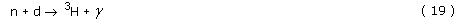eventually producing 4He in the reaction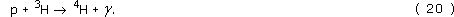In their universe, the increase of neutron lifetime will mean that at any point in time there will be more neutrons in their universe than in ours. Moreover, the ratio of neutrons in their universe to neutrons in ours will steadily increase with time. Thus although at the time when Reaction 15 is important their rate of formation of deuterium will be somewhat faster than ours, by the time the later Reaction 19 comes into its own their rate of destroying deuterium will be greater still in comparison with ours. Thus, deuterium will be formed more readily, but destroyed even more readily. The net result will be that they find less deuterium than we do.

Figure 32 shows how the computed freeze-out mass fractions of light nuclei depend on the assumed present-day value of the baryonic density. As you can see, the freeze-out abundance of 4He is almost independent of the present baryonic density, whereas the freeze-out abundance of deuterium 2H depends very sensitively on ρb,now. The most abundant nuclide of hydrogen (1H) is not shown, but if it had been shown, it too would have been relatively insensitive to the present baryonic density, its mass fraction being about 0.75.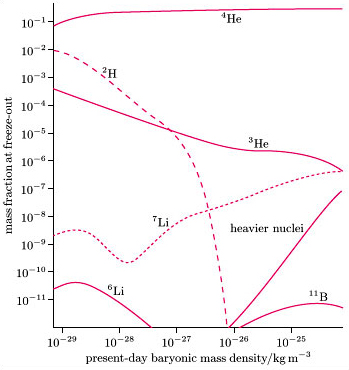adapted from R.V. Wagoner (1973) The Astrophys. J., 179(2), 349
Figure 32 Predicted freeze-out mass fractions (at around 104 s after the big bang) for various light nuclei plotted against the assumed present-day mass density of baryonic matter, ρb,now/c2
S357_1